New Analog Processing Technique in Multichannel Neural Signal Recording with Reduce Data Rate and Reduce Power Consumption

New Analog Processing Technique in Multichannel Neural Signal Recording with Reduce Data Rate and Reduce Power Consumption

Department of Electrical and Computer Engineering, Tarbiat Moallem University, Sabzevar, Iran

Corresponding Author Email:
Page:
133-137
|
DOI:
https://doi.org/10.18280/ts.360202
9 January 2019
|
Accepted:
22 March 2019
|
Published:
5 July 2019
| Citation

OPEN ACCESS

Abstract:

Nowadays, the design of multichannel recording systems for neural signals used as an irreplaceable tool in the storage and analysis of neural signals for the diagnosis and treatment of various cardiovascular diseases. In order to increase the information received and thus reduce the risk of using these sorts of systems, designers try to use more electrodes or channels in this systems, but if the number of channels increases the new constraint is added to these systems which is stores a vast amount of data, and makes a wireless transport of information impossible. So it causes an increase in the number of channels in signal recording systems are severely restricted. The purpose of this study is to create a new structure for the analog processors to we do not only transfer the stored spikes completely to the system output but also reduce the amount of information which need to transmit. This new method consists of two separate compressive sampling blocks and spike detecting which is implanting together. Through this study, it was found that by using this method, we can increase the channel of neural signal recording system without any limitation, So The findings of this research significantly decrease the risk of using neural signal recording systems for biomedical applications.

Keywords:

analog processor, compressive sampling, spike detection, multi-channel neural recording system, reduce power consumption

1. Introduction

THE Brain Machine Interface is the case of many neural signals analyzes since 1970, which divides it into EEG, MEG, neural recording, ECoG, fMRI, and NIRS based on the method of storing signals. Among the above methods, implantable systems of neural signal recording are one of the most important parts of the BMI that have the best performance for understanding brain function . The typical multichannel neural signal recording system is designed as follows. 

1.png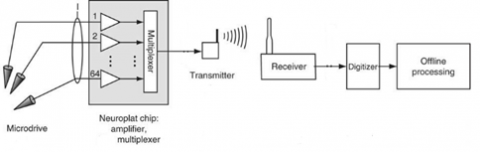Figure 1. Typical multi-channel neural recording system

Generally, in the designing of the neural signals recording system, researchers care about the design of the above blocks such as LNA, Filters, and ADC. In order to increase the received data and so reducing the risk of using these types of systems, designers every day try to use more electrodes or channels in the neural signal recording system. For example, the largest number of designed channels is 11011, which transmitted the collected data via wire . However, this kind of research indicates the importance of increasing the number of channels for the neural signal recording system.

But if the number of channels increases, in addition to past constraints, such as power consumption, noise, and size, a new limitation is added to these systems which are a large amount of data stored, so make it impossible to transmit data in wireless. Therefore the number of channels in neural signal recording systems will severely be restricted. Also, by increasing the complexity and the size of the information collected, we need to combine and process the collected data, which makes the neural signal processor as an important part of the neural signal recording systems. Neural signals processing achieved in both digital and analog forms and each of them holds its own disadvantages and advantages. Our goal is to provide a simple, easy-to-implement and low-cost system, therefore, we can use the analog signal processing system as shown in Figure 2.

2.pngFigure 2. The location of the analog and digital processor in the neural signal recording system

In this systems for transferring stored data, designers need to reduce the number of extracted data, in such a way the quality of the original signal is not reduced. To solve this problem, two general solutions suggested.

1- Spike Detection

2- Signal Compression

In this article, we used both of the above methods to process stored signals and reduce the data sent.

The remainder of this paper is organized as follows: Section 2 introduces Spike detecting and how we designed our spike detector, Section 3 describes Signal compression method and in Section 4 demonstrates how we used this methods to make new way for analog processing.

2. Spike Detecting

There is still no final agreement between neurology researchers on how to decode intra-brain information. One theory suggests that information coding performed by the action Potential signal frequencies [3-4]. Another theory suggests that the action potential timing determines information in the neural signals [4-5]. Regardless of which of the above two theories is correct, there is no doubt that the essential part of all the bioelectric signals is the Action Potential (AP).

Spike detection circuits designed with different algorithms. In most of the designs, Spike detected first, and the detector circuit displays the pulse as a spike detection in the output. The output of this type of Spike detector shown in Figure 3 [6-8]. In fact, in this way, a great deal of unnecessary information is eliminated, but the problem is that the use of this method results in the loss of some crucial information. Using this technique is only valuable for researchers who need to detect the spike and the time the spike accrued, with the output only pulsed. But for some specific diseases, as well as when all the data in the neural signal is required to process, this method will not work.

3.png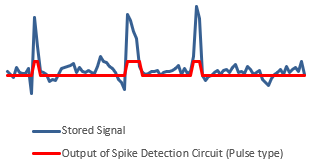Figure 3. The blue diagram shows the stored signal, and the red diagram shows the output of the Spike detection circuit

4.pngFigure 4. Schematic spike detector circuit with spike output in the technology of 180 nm

To solve the above problem, we have proposed a solution for the Spike detection circuit which is spike detector depict, Spike itself, instead of the pulse. In this method, the output is spikes rather than pulses. By using this method, not only a large part of the surplus information is removed but also all of the saved signals sent to the output, so no vital information removed from our stored signal. For this purpose, in Figure 4, a comparator and capacitor switches have been used. In our design, we used 180nm technology.

The output of our spike detection circuit is not a pulse, and all stored spikes transmitted to the output. In Figure 5, the output of the above circuit shown.

5.png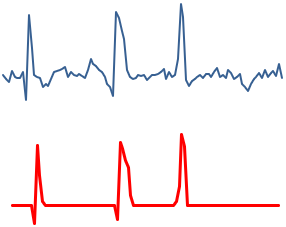Figure 5. The blue diagram is the stored signal and the red diagram shows the output of the Spike detection circuit, which are Spikes according to the use of the capacitor switches

Table 1. Reduced data sent in both pulse type and spike type spike detection methods

 Spike detection Percentage of data reduction Pulse type 75 % Spike type 35 %

Table 1 illustrate the percentages of data reduction in each of the two methods.

As shown in Table 1, the amount of data reduction in Pulse Type method is far more than the Spike Type method. However, as we mentioned before, due to the Pulse Type method limitation, which cannot transmit some critical part of the stored signal, so reliability in this method is low. And the compressive sampling circuit after the Spike detection circuit with pulse output is not suitable. But using the Spike Type method not only we can transmit all vital information of the stored signal, it also has the ability to reduce the amount of data by compressing it.

3. Signal Compression

The traditional method of recovering signals and images from measured data is based on the Shannon- Nyquist sampling theory, according to this method, the minimum number of samples which is required for sampling to recover the main signal without missing is twice the maximum frequency of the main signal.

This theory is the foundation of most of the existing technology equipment, including analog-to-digital converters, medical imaging devices, or electronics equipment. The Compression Sampling (CS) theory which also referred to as "Compressive Sensing" is basically a new method to provide data collection and replace the Shannon method.

This theory claims that a sparse signal can recover with a number of samples much fewer than Nyquist rate. In this method first, we compress received neural signals by using mathematical equation. And at the end of the process, we can recover signals by using reconstruction algorithms. So, all the necessary information of the neural signals can be obtained. This improvement led to the higher approval of this method in recent studies [9-10].

In fact, the theory of CS in order to obtain a vector of samples y ɛ Rn , for each sparse signal x ɛ Rm, is a sparse transformation matrix Ψ ε Rm, m, and the random matrix Φ ε Rn, m  is employed. In mathematical terms:

$y_{n * 1}=\Phi_{n * m} x_{m * 1}=\Phi_{n * m} \Psi_{m * m} \theta_{m * 1}=A_{n * m} \theta_{m * 1}$ (1)

That $\theta=x \Psi^{-1}$ is sparse represent of x. Assuming that the signal x, -K is sparse, x is a vector with the maximum k non-zero array. We seem to face the lower-order equation for recovering the vector x and y, because the number of equations (n) is less than the number of variations (m). Therefore, there will be the endless answer .but with the initial knowledge about the sparsity of the signal in many cases, it can be possible to get some answers .

Compressive sampling is one of the new methods for compression of signals, which has a good potential for compression of neural signals [12, 13]. The only condition for using this method is the sparsity of the primary signal, meaning that most of the information in the signal is dispersed in discrete sections of the signal waveform, although the neural signals are Sparse, and there is no problem with using this method for compressing neural signals.

Figure 6 describes how this method works . We initially translate the signal to the sparse state using the matrix Φ, then we compress our main signal using the (Sensing matrix) compression random matrix Ψ [12, 15]. And after the transition of the signal, our main signal is recovered [16, 17].

6.png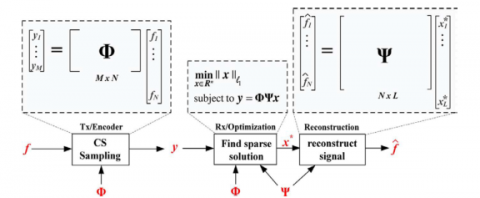Figure 6. Function of compression sampling technique

Some examples of using this technique in analog circuits is Random Convulsion , Random Filters  and Compressive multiplexers .

We used Figure 7 structure to design analog compressive sampling.

7.pngFigure 7. The system used to compress the analog signal using the compression sampling technique

In this structure, the signal is first modulated randomly by r(t). This system is consists of a random number generator (PC), mixer, accumulator and sampler, although the sampler used here does not need sampling according to the Nyquist rate. In fact, the advantage of this method is the use of sampling at a much lower rate than the Nyquist rate.

8.png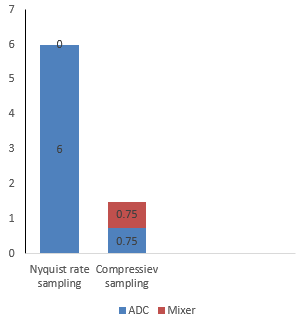Figure 8. Comparison of power consumption of compressive sampling and sampling at Nyquist rate

In order to check the power consumption of the compressive sampling systems and use of ADCs at the Nyquist rate, we compare their energy consumption in Figure 8. For Nyquist rate sampling, the measured power consumption is the power consumption of the analog to digital converter, but in the case of using compressive sampling method, there is also a mixer in addition to the analog-to-digital converter. This value is measured for a time period of one second and the 64-channel signal recording system .

As you can see, with using the compressive sampling systems, the total power consumption of analog to digital converters and mixers can reduce power consumption by 75 %. The above results also indicate the suitability of using analog circuits, for designing compressive sampler.

4. Analog Processor

In fact, the designed analog processor combines the use of Spike Detection with Spike Output mode and Compressive Sampling Circuits method. There is a trade-off between increasing complexity of the system and reducing the power consumption.

The block diagram of our designed neural signals recording system is as follows.

9.pngFigure 9. Neural signal recording block diagram using spike detection and compressive sampling

In fact, 3 intermediate blocks, including the Spike Detection Block, Compressive Sampling and the Analog to Digital Converter, are called Analog to Information Converters. The designed analog processor system reduce about 40 % of amount of data in the Spike detection block, and reduce about 75% of the data in the compressive sampling block, which in total, using the combination of the two methods, reduces about 85% of the sent data, which respectably the power consumption of our system will be reduced.

By comparing the various methods of analog processing and reducing the data provided by them in Table 2, we find that in the method used in this paper, however, the use of two methods simultaneously to reduce data complicates the system, but it reduces the sent data up to 15 %, which is very remarkable.

Table 2. The percentage of data reduction range by using different data reduction techniques

 Data reduction technique Data Reduction Pulse type - spike detection 75% - 80% Spike type – spike detection 35% - 45% Compressive sampling 50% - 85% This project method 85% - 90%
5. Conclusions

In this paper, a new method for the analog processing of data proposed for neural signal recording systems, which is a combination of spike detection techniques and compressive sampling. And we compared it with the verity of data reduction techniques. Our method is to reduce about 85% of the sent data, but vital data that is essential for the neural signal recording system, completely transmitted.

The risk of using our method is significantly lower for use in biomedical applications. This data reduction in the first stage will reduce the power consumption and will also allow to the multiplication of the number of channels of the neural signal recording system.

In the next step there is two important way to continue this research the first one is improving compressing sampling algorithm to optimize this method and also we could research about the efficiency of this method for different number of electrodes.

References

 Gosselin, B., Sawan, M. (2010). A low-power integrated neural interface with digital spike detection and extraction. Analog Integrated Circuits and Signal Processing, 64(1): 3-11. http://dx.doi.org/10.1007/s10470-009-9371-1

 Frey, U., Egert, U., Heer, F., Hafizovic, S., Hierlemann, A. (2009). Microelectronic system for high-resolution mapping of extracellular electric fields applied to brain slices. Biosensors and Bioelectronics, 24(7): 2191-2198. http://dx.doi.org/10.1016/j.bios.2008.11.028

 Adrian, E.D., Zotterman, Y. (1986). The impulses produced by sensory nerve endings, Part 2. The response of a single end-organ. The Journal of Physiology, 61: 151–171. http://dx.doi.org/10.1113/jphysiol.1926.sp002281

 Perkel, D.H., Bullock, T.H. (1968). Neural coding. Neurosciences Res. Prog. Bull., 6(3): 221–348.

 Hopﬁeld, J.J. (1995). Pattern recognition computation using action potential timing for stimulus representation. Nature, 376(6535): 33–36. http://dx.doi.org/10.1038/376033a0

 Semmaoui, H., Drolet, J., Lakhssassi, A., Sawan, M. (2012). Setting adaptive spike detection threshold for smoothed TEO based on robust statistics theory. IEEE Transactions on Biomedical Engineering, 59(2): 474-482. http://dx.doi.org/10.1109/TBME.2011.2174992

 Chan, H.L., Lin, M.A., Wu, T., Lee, S.T., Tsai, Y.T., Chao, P.K. (2008). Detection of neuronal spikes using an adaptive threshold based on the max–min spread sorting method. Journal of Neuroscience Methods, 172(1): 112-121. http://doi.org/10.1016/j.jneumeth.2008.04.014

 Thakur, P.H., Lu, H.Z., Hsiao, S.S., Johnson, K.O. (2007). Automated optimal detection and classification of neural action potentials in extra-cellular recordings. Journal of Neuroscience Methods, 162(1-2): 364-376. http://dx.doi.org/10.1016/j.jneumeth.2007.01.023

 Shoaib, M., Jha, N.K., Verma, N. (2015). Signal processing with direct computations on compressively sensed data. IEEE Transactions on Very Large Scale Integration (VLSI) Systems, 23: 30-43. http://dx.doi.org/10.1109/TVLSI.2014.2301733

 He, B.D., Wein, A., Varshney, L.R., Kusuma, J., Richardson, A.G., Srinivasan, L. (2015). Generalized analog thresholding for spike acquisition at ultra-low sampling rates. Journal of Neurophysiology, 114(1): 746-760. https://doi.org/10.1152/jn.00623.2014

 Li, Y.Q., Yu, Z.L., Bi, N., Xu, Y., Gu, Z.H., Amari, S. (2014). Sparse representation for brain signal processing: A tutorial on methods and applications. IEEE Signal Processing Magazine, 31(3), 96106. http://dx.doi.org/10.1109/MSP.2013.2296790

 Eldar, Y.C., Kutyniok, G. (2012). Compressed Sensing: Theory and Applications. Cambridge University Press, Cambridge UK, 1-303. http://dx.doi.org/10.1017/CBO9780511794308

 Peyre, G. (2010). Best basis compressed sensing. IEEE Transactions on Signal Processing, 58: 2613-2622. http://dx.doi.org/10.1109/TSP.2010.2042490

 Chen, F., Chandrakasan, A.P., Stojanovic, V.M. (2012). Design and analysis of a hardware-efficient compressed sensing architecture for data compression in wireless sensors. The IEEE Journal of Solid-State Circuits, 47(3): 744-756. http://dx.doi.org/10.1109/JSSC.2011.2179451

 Dimakis, A.G., Smarandache, R., Vontobel, P.O. (2012). LDPC codes for compressed sensing. IEEE Transactions on Information Theory, 58: 3093-3114. http://dx.doi.org/10.1109/TIT.2011.2181819

 Candes, E.J., Tao, T. (2005). Decoding by linear programming. IEEE Transactions on Information Theory, 51(12): 4203-4215. http://dx.doi.org/10.1109/TIT.2005.858979

 Rudelson, M., Vershynin, R. (2006). Sparse reconstruction by convex relaxation: Fourier and Gaussian measurements. 2006 40th Annual Conference on Information Sciences and Systems. http://dx.doi.org/10.1109/CISS.2006.286463

 Mamaghanian, H., Khaled, N., Atienza, D., Vandergheynst, P. (2012). Design and exploration of low-power analog to information conversion based on compressed sensing. IEEE Journal on Emerging and Selected Topics in Circuits and Systems, 2(3): 493-501. http://dx.doi.org/10.1109/JETCAS.2012.2220253

 Tropp, J.A., Wakin, M.B., Duarte, M.F., Baron, D., Baraniuk, R.G. (2006). Random filters for compressive sampling and reconstruction. 2006 IEEE International Conference on Acoustics, Speech, and Signal Processing, 3: 872-875. http://dx.doi.org/10.1109/ICASSP.2006.1660793

 Slavinsky, J.P., Laska, J.N., Davenport, M.A., Baraniuk, R. (2011). The compressive multiplexer for multi-channel compressive sensing. Proceedings of the IEEE International Conference on Acoustics, Speech and Signal Processing, ICASSP, pp. 3980-3983. http://dx.doi.org/10.1109/ICASSP.2011.5947224